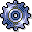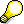Template for Formula Planning ProcessUse

Create templates for the formula planning of business processes in the BPP environment.

You can choose between five row types. Depending on the row type you choose, the following columns are available for data entry:

 Row Type Available columns Commentary Row Name/Item text Sub-Template Object, Name, Activation condition Statistical Key Figure (StKF) Object, Name, Quantity fixed formula, Quantity variable/ServUn formula, Activation condition Object, Name, Quantity fixed formula, Activation conditions Cost Element Object, Name, Costs fixed formula, Costs variable/ServUn formula, Quantity fixed formula, Quantity variable/ServUn formula, Activation conditions

You have the following entry and maintenance possibility per column type.

 Column type Possible Entries/Processing Row Type Commentary line, Sub-template, Statistical key figure, Calculation row, Cost element Object According to the row type: cost element, statistical key figure, and so on Name Descriptive name for the line Costs fixed formula fixed costs You can specify these values as constants, or you can have the system calculate them dynamically at during evaluation - the corresponding editor is available (for procedures on the Editor, see: Quantity Determination). Costs variable/LE Formula Variable costs Quantity fixed formula Fixed quantity Quantity variable/LE Formula Variable quantity Activation Requirements You can enter Active or Inactive as fixed values, or you can define a method which allows the R/3 System to activate or deactivate the item when the valuation is run (see Activation).If you choose the row type calculation row, the editor cannot be called up in the object columns.

For more information on creating a template, see Editing Templates.